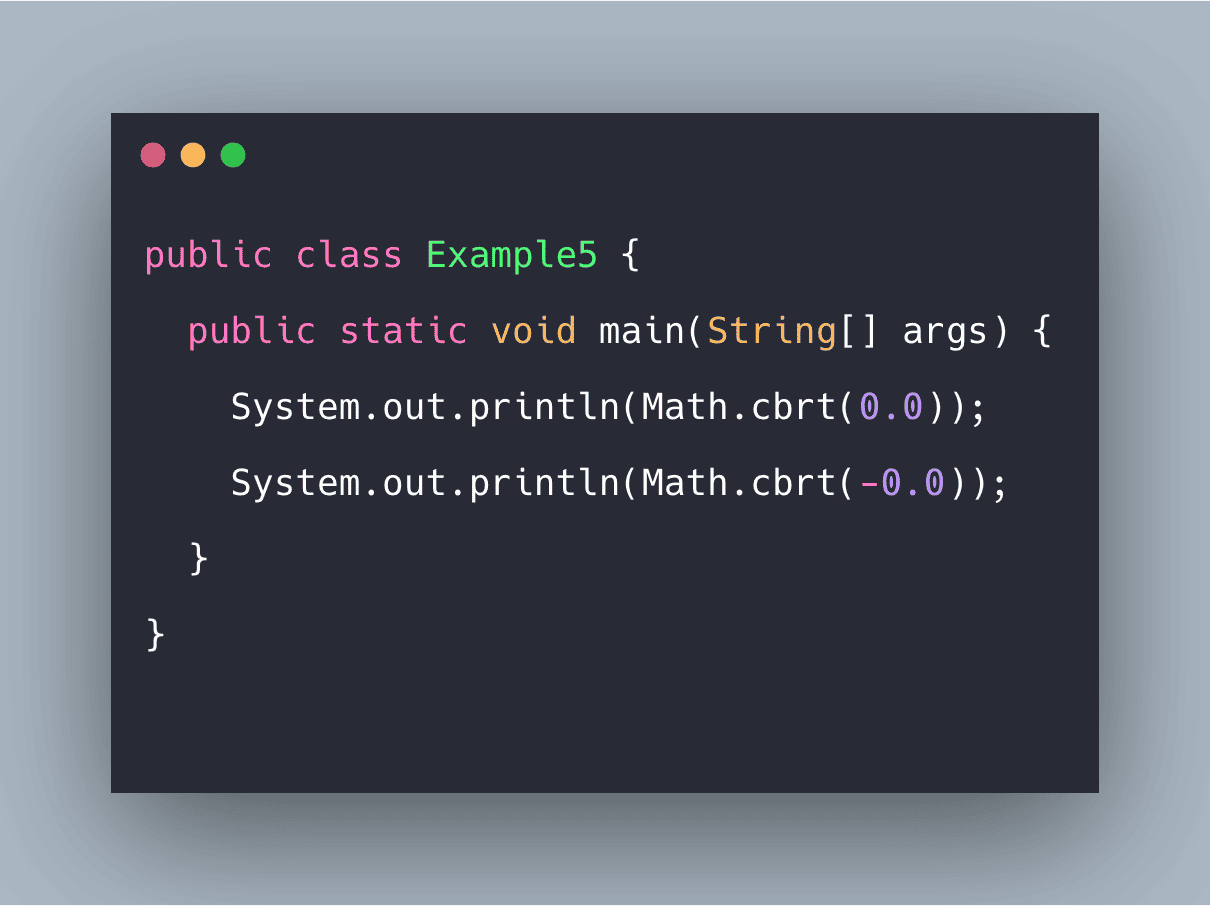# Java Math cbrt() Function ExampleJava.lang.Math.cbrt() is an inbuilt method that is used to calculate the cube root of any number (passed as double type).

Java.lang.Math.cbrt() is an inbuilt method that is used to calculate the cube root of any number (passed as double type). The cbrt() method can come handy in several programs involving mathematical calculations. As defined by Java(Oracle), the computed result for this method must be within 1 ulp(unit of least precision of the exact result.

### Java Math cbrt()

The java.lang.Math.cbrt(double a) returns the cube root of a double value. For positive finite x, cbrt(-x) == -cbrt(x); that is, the cube root of a negative value is the negative of the cube root of that value’s magnitude.

#### Syntax

``public static double cbrt(double x)``

## How to Install OpenJDK 11 on CentOS 8

What is OpenJDK? OpenJDk or Open Java Development Kit is a free, open-source framework of the Java Platform, Standard Edition (or Java SE).

## Math class in Java Example | Java Math Class Tutorial

Math class in Java Example. Using the Math class, we can implement methods like min(), max(), avg(), sin(), cos(), tan(), round(), ceil(), floor(), abs(), pow(), floor(), ciel(), round()

## Java Online Training | Java Online Course | ITGuru

Our Java Online Training provide you to learn about Java programming and its different features with realty. Our Java Online Course includes live sessions, live projects

## Best Java IDE 2020 | Most Popular Java IDE

Our Java Online Training provide you to learn about Java programming and its different features with realty. Our Java Online Course includes live sessions, live projects

## Learning by Doing: How to Learn Java Basics by Building Your Own Project

Check out some ideas for sample projects that can help you learn Java, and programming in general, in a fun and engaging way.